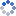加载中…疾风劲草心儿
• 博客等级：• 博客积分：0
• 博客访问：70,698
• 关注人气：3
• 获赠金笔：0支
• 赠出金笔：0支
• 荣誉徽章：

## Python数据正弦拟合

(2015-09-21 23:34:58)

### 正弦振幅

import numpy as np
from scipy.optimize import leastsq
import pylab as plt

N = 1000 # number of data points
t = np.linspace(0, 4*np.pi, N)
data = 3.0*np.sin(t+0.001) + 0.5 + np.random.randn(N) # create artificial data with noise

guess_mean = np.mean(data)
guess_std = 3*np.std(data)/(2**0.5)
guess_phase = 0

# we'll use this to plot our first estimate. This might already be good enough for you
data_first_guess = guess_std*np.sin(t+guess_phase) + guess_mean

# Define the function to optimize, in this case, we want to minimize the difference
# between the actual data and our "guessed" parameters
optimize_func = lambda x: x*np.sin(t+x) + x - data
est_std, est_phase, est_mean = leastsq(optimize_func, [guess_std, guess_phase, guess_mean])

# recreate the fitted curve using the optimized parameters
data_fit = est_std*np.sin(t+est_phase) + est_mean

plt.plot(data, '.')
plt.plot(data_fit, label='after fitting')
plt.plot(data_first_guess, label='first guess')
plt.legend()
plt.show()

0